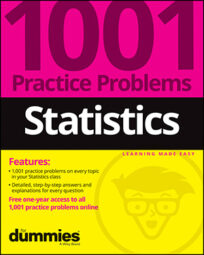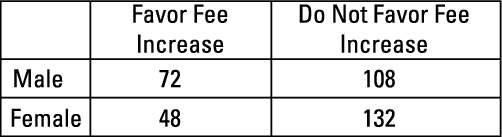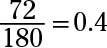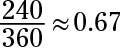##### Statistics: 1001 Practice Problems For Dummies (+ Free Online Practice)Two-way tables are used to organize and interpret pairs of categorical variables. Here, you practice reading and interpreting all parts of a two-way table. This 2-x-2 table displays results from a poll of randomly selected male and female college students at a certain college, asking whether they were in favor of increasing student fees to expand the college's athletics program.

The results of their opinions are broken down by gender in the following table.Credit: Illustration by Ryan Sneed

## Sample questions

1. What does the value 72 represent in this table?

Answer: the number of all students polled who are both male and favor an increase

In a cross-tabulation table, the number in an individual cell represents the cases that have the characteristics described by the row and column intersecting at that cell. In this example, 72 is in the cell at the intersection of the row for Male and the column for Favor Fee Increase so 72 represents the number of students who are both male and favor a fee increase.

2. How many of the students are female and favor the fee increase?

To find the number of students polled who are both female and favor the fee increase, find the cell where the row for Female and the column for Favor Fee Increase intersect. The value in this cell is 48. The keyword in this question is and, which means intersection.

3. How many female students were included in this poll?

To find the total number of female students included in this poll (out of all 360 students polled), add the values in the row labeled Female: 48 + 132 = 180.

This sum represents all the students who are female and in favor of the increase plus all the students who are female and not in favor of the increase.

4. How many students do not favor the fee increase?

To find the total number of students who don't favor the fee increase, add the values in the column labeled Do Not Favor Fee Increase: 108 + 132 = 240.

This sum represents all the students who are not in favor of the increase and male plus all the students who are not in favor of the increase and female.

5. What proportion of male students favors the fee increase?

A proportion involves a fraction, including a numerator and denominator. The denominator is the key because it represents the total number of individuals in the group you're looking at, and you may be looking at different groups from problem to problem.

To find the proportion of male students who favor the fee increase, you divide the number of students who are male and in favor of the fee increase (72) by the total number of male students in the poll (72 + 108 = 180):Note that you don't divide by 360 (all students polled) because the question asks you to find the proportion of male students, not both male and female students, who favor the increase. So you divide by the total number of male students polled (180).

6. What proportion of all students does not favor the fee increase?

A proportion involves a fraction, including a numerator and denominator. The denominator is the key because it represents the total number of individuals in the group you're looking at, and you may be looking at different groups from problem to problem.

To find the proportion of all students who don't favor the fee increase, divide the total number of students who don't favor the fee increase (108 + 132 = 240) by the total number of students in the poll (108 + 132 + 72 + 48 = 360):Note that you divide by 360 here because you want the proportion of all students.

If you need more practice on this and other topics from your statistics course, visit 1,001 Statistics Practice Problems For Dummies to purchase online access to 1,001 statistics practice problems! We can help you track your performance, see where you need to study, and create customized problem sets to master your stats skills.